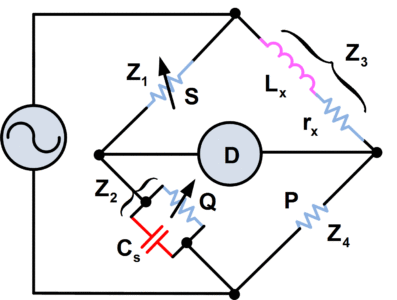Home / Instrumentation / Maxwell Inductance Bridge Circuit

# Maxwell Inductance Bridge Circuit

Want create site? Find Free WordPress Themes and plugins.

For measurement of inductance, the Maxwell Bridge shown in figure 1 can be employed. It is seen that the circuit of the Maxwell Bridge is simply a repeat of the series resistance-capacitance bridge, with the capacitors replaced by the inductors.Fig.1: Maxwell Bridge

A disadvantage of this bridge is that the standard inductors are larger and difficult to manufacture than standard capacitors. Consequently, a variation of this circuit, known as the Maxwell-Wein Bridge, is most often employed for inductance measurement.

The circuit of the Maxwell-Wein Bridge is shown in figure 2.Fig.2: Maxwell-Wein Bridge

Lx is the unknown inductance to be measured, and rx is the resistance of its windings. Cs is again a precise standard capacitor, and P is a standard resistor. Q and S are accurately adjustable resistors.

## Maxwell Bridge Working Principle

At balance, we have the following equation:

$\frac{{{Z}_{1}}}{{{Z}_{2}}}=\frac{{{Z}_{3}}}{{{Z}_{4}}}$

Therefore,

$\begin{matrix} \frac{S}{{}^{1}/{}_{(\frac{1}{Q}+j\omega {{C}_{s}})}}=\frac{{{r}_{x}}+j\omega {{L}_{x}}}{P} & \cdots & (1) \\\end{matrix}$

Or

$S(\frac{1}{Q}+j\omega {{C}_{s}})=\frac{{{r}_{x}}+j\omega {{L}_{x}}}{P}$

$\begin{matrix} \frac{S}{Q}+j\omega {{C}_{s}}S=\frac{{{r}_{x}}}{P}+\frac{j\omega {{L}_{x}}}{P} & \cdots & (2) \\\end{matrix}$

Equating the real and imaginary terms:

$\begin{matrix} \frac{S}{Q}=\frac{{{r}_{x}}}{P} & \cdots & (3) \\\end{matrix}$

And

$\omega {{C}_{s}}S=\frac{\omega {{L}_{x}}}{P}$

Formula for Unknown Inductance$\begin{matrix} {{L}_{x}}=P{{C}_{s}}S & \cdots & (4) \\\end{matrix}$

Once again it is seen that the supply voltage and the frequency are not involved in the balance equations for the bridge. This is not always the case with AC bridges; indeed, one particular bridge can be used to measure the frequency of the supply in terms of the bridge components values at balance.

Did you find apk for android? You can find new Free Android Games and apps.